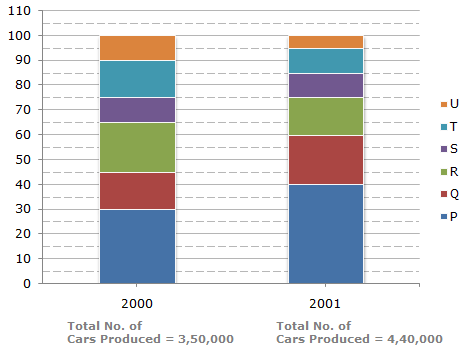# Data Interpretation - Bar Charts - Discussion

Discussion Forum : Bar Charts - Bar Chart 9 (Q.No. 2)
Directions to Solve

The bar graph given below shows the percentage distribution of the total production of a car manufacturing company into various models over two years.

Percentage of Six different types of Cars manufactured by a Company over Two Years2.
Total number of cars of models P, Q and T manufactured in 2000 is?
2,45,000
2,27,500
2,10,000
1,92,500
Explanation:

Analysis of the graph:

We shall first determine the number of cars of each model produced by the Company during the two years:

In 2000 : Total number of cars produced = 3,50,000.

P = (30 - 0)% of 3,50,000 = 30% of 3,50,000 = 1,05,000.

Q = (45 - 30)% of 3,50,000 = 15% of 3,50,000 = 52,500.

R = (65 - 45)% of 3,50,000 = 20% of 3,50,000 = 70,000.

S = (75 - 65)% of 3,50,000 = 10% of 3,50,000 = 35,000.

T = (90 - 75)% of 3,50,000 = 15% of 3,50,000 = 52,500.

U = (100 - 90)% of 3,50,000 = 10% of 3,50,000 = 35,000.

In 2001 : Total number of cars produced = 4,40,000.

P = (40 - 0)% of 4,40,000 = 40% of 4,40,000 = 1,76,000.

Q = (60 - 40)% of 4,40,000 = 20% of 4,40,000 = 88,000.

R = (75 - 60)% of 4,40,000 = 15% of 4,40,000 = 66,000.

S = (85 - 75)% of 4,40,000 = 10% of 4,40,000 = 44,000.

T = (95 - 85)% of 4,40,000 = 10% of 4,40,000 = 44,000.

U = (100 - 95)% of 4,40,000 = 5% of 4,40,000 = 22,000.

Total number of cars of models P, Q and T manufacture in 2000

= (105000 + 52500 + 52500)

= 2,10,000.

Discussion:
6 comments Page 1 of 1.

Deepa G S said:   9 months ago
P+Q+T = 60%.

60% of 3,50,000 = 2,10,000 Ans.

Chetanpreet said:   7 years ago
Simple, 60%of 3,50,000 = 2,10,000.

Ashish Jalit said:   1 decade ago
Total no.of car model = P+Q+R.

30+(45-30)+(90-75) = 30+15+15 = 60.

Total no.of car produce = 3,50,000.

No.of car produce = 3,50,000/100 = 3,500.

Therefore total no. of car model = 60*3,500 = 2,10,000
(1)

That's simple

p=30, q=15, t=15

(30+15+15)% of 3,50,000

60*3,50,000/100 = 2,10,000.